# Homework H3.A - Fa23

 Problem statement Solution video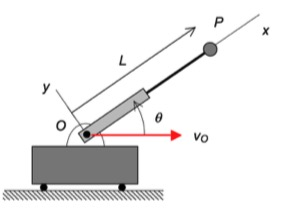DISCUSSION and HINTS
As can be seen from the animation below, the path taken by P is a bit complex. However, what if we put an observer on the non-extending section of the rotating arm - what motion would the observer see for P? Think about that.Suppose that we do put an observer on the non-extending portion of the rotating arm. That observer would describe the motion of P as being on a straight path aligned with the x-axis. Specifically, we have:

(vP/O)rel = L_dot i
(aP/O)rel = L_ddot i

Using this observer, we can write down the velocity and acceleration of P using the moving reference frame velocity and acceleration equations:

vP = vO + (vP/O)rel + ω x rP/O
aP = aO + (aP/O)rel + α x rP/O + 2ω x (vP/O)rel + ω x (ω x rP/O)

where ω and α are the angular velocity and angular acceleration, respectively, of the observer.

# Homework H3.B - Fa23

 Problem statement Solution video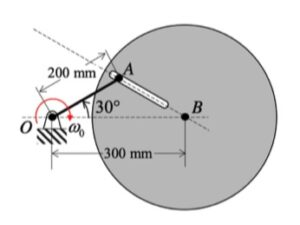DISCUSSION and HINTS
In this problem, we desire to relate the rotation rates of link OA and the disk.With the two rigid bodies connected by a pin-in-slot joint, we are not able to use the previous rigid body kinematics equations by themselves. Let's discuss that below.

Velocity analysis
Here, we can use the rigid body velocity equation to relate the motions of O and A:

vA = vO + ωOA x rA/O

However, we cannot use a rigid body velocity equation to relate the motion of points A and B (the reason for this is that A and B are not connected by a rigid body). In its place, we can use the moving reference frame velocity equation with an observer attached to the disk:

vA = vB + (vA/B)rel + ω x rA/B

where ω is the angular velocity of the observer, and (vA/B)rel  is the velocity of A as seen by our observer on the disk. Note that with the observer being attached to the disk, this observer sees motion of A only along the slot.

Combine these two equations to produce two scalar equations.

Acceleration analysis
We will use the same procedure for acceleration as we did for velocity - use a rigid body equation for OA and a moving reference frame equation relating A and B.

aA = aO + αAOx rA/O + ωAO x (ωAO x rA/O)
aA = aB + (aA/B)rel + α x rA/B + 2ω x (vA/B)rel + ω x (ω x rA/B)

where α is the angular acceleration of the observer, and (aA/B)rel  is the acceleration of A as seen by our observer on the disk. Again, note that with the observer being attached to the disk, this observer sees motion of A only along the slot.

Combine these two equations to produce two scalar equations.

# Homework H2.I - Fa23

 Problem statement Solution videoDISCUSSION
Suggested solution strategy:

• vA = vO + ωOA x rA/O
• vB = vH + ωBH x rB/H
• vA = vB + ωAB x rA/B
• Combine above, solving for the two unknown angular velocities.
• vE = vB + ωAB x rE/B# Homework H2.J - Fa23

 Problem statement Solution videoDISCUSSION
Suggested solution strategy:

• vB = vE + ωBE x rB/E
• vA = vO + ωAO x rA/O
• vA = vB + ωAB x rA/B
• Combine above, solving for the two unknown angular velocities.
• Repeat the above for accelerations.# Homework H2.G - Fa23

 Problem statement Solution video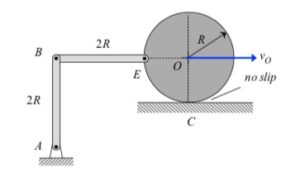DISCUSSION

• The no-slip point C is the IC for the disk. Therefore, the velocity of E, vE, is perpendicular to the line EC.
• AB rotates about the fixed point A. Therefore, the velocity of B, vB, is perpendicular to line AB.
• Construct perpendiculars to vE and vB.
• The intersection of these two perpendiculars is the IC for BE.In a freeze-frame of the simulation results below, note that vE is perpendicular to line EC, as expected.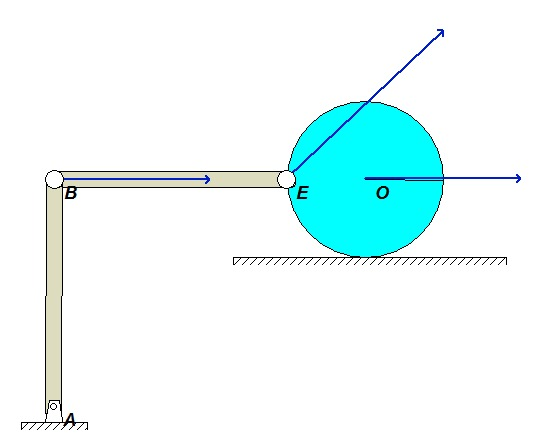# Homework H2.H - Fa23

 Problem statement Solution videoDISCUSSION
Suggested solution strategy:

• Find IC for DB at the intersections of the velocities for D and B. Call this point K. In which direction is link DB rotating? And, in which direction is AB rotating?
• Find IC for DG at the intersections of the velocities for D and G. Call this point N. In which direction is link DG rotating? And, in which direction is EG rotating?
• Find IC for GH at the intersections of the velocities for G and H. In which direction is GH rotating?In a freeze-frame of the simulation results below, note the direction of motion for points D, B, G, E and H. Do these directions agree with those found in your analysis?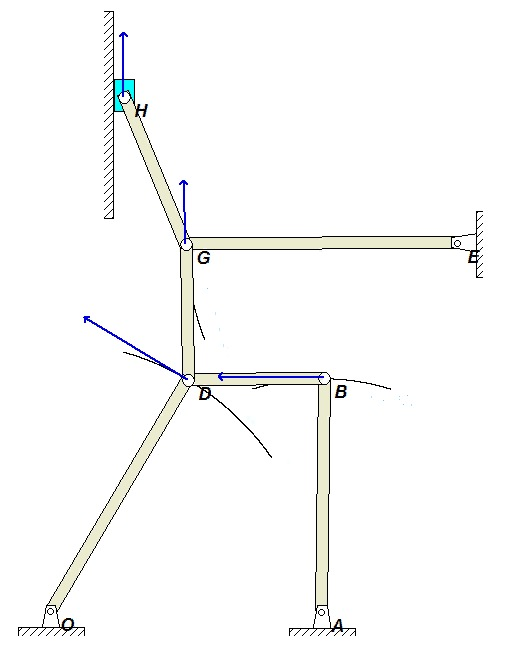# Homework H2.E - Fa23

 Problem statement Solution videoDISCUSSION
For velocity analysis, you will need a rigid body velocity equation for the triangular plate:

vA = vB + ωAB x rA/B

Take note that A and B both have constrained motion along their respective slots.# Homework H2.F - Fa23

 Problem statement Solution videoDISCUSSION
For velocity analysis, you will need a rigid body velocity equation for each link:
vB = vO + ωOB x rB/O
vA = vB + ωAB x rA/B

For acceleration analysis, you will need a rigid body acceleration equation for each link:
aB = aO + αOB x rB/OωOB2 rB/O
aA = aB + αAB x rA/B - ωAB2 rA/B

Take note that A has constrained motion along the inclined slot.# Homework H2.C - Fa23

 Problem statement Solution videoDISCUSSION
The tank is known to roll without slipping on the bed of the truck, with both the truck and the center of the tank C moving with constant speeds. Let D represent the contact point of the tank on the bed of the truck. As you write down the acceleration equation for point A referenced to the no-slip point D:
aA = aDα x rA/D - ω2rA/D
do not forget that the contact point D has a vertical component of acceleration.

# Homework H2.D - Fa23

 Problem statement Solution videoDISCUSSION
For velocity analysis, you will need a rigid body velocity equation for each link:
vA = vB + ωAB x rA/B
vA = vC + ω
disk x rA/C
and, for acceleration analysis you will need a rigid body acceleration equation for each link:
aA = aBαABx rA/B- ωAB2rA/B
aA = aC + α
disk x rA/C - ωdisk2rA/C
In addition for acceleration, you also need a rigid body acceleration equation relating points O and C on the disk.